Question Pool Second Degree Equations

# Second Degree Equations - SAMAGRA Question Pool & Answers | Class 10 English Medium

Kerala Syllabus SAMAGRA SCERT SAMAGRA Question Pool for Class 10 English Medium Maths Second Degree EquationsQn 1.

Length of a rectangle is 10 cm more than the breadth. If the area is 144 square cm, find the length and breadth of the rectangle.

Get Free Study Materials + 1 Week Free Trial of BrainsPrep Class 10 English Medium Tuition

Qn 2.

Difference between  two numbers is 4 and its product is 96. Find the numbers.

Get Free Study Materials + 1 Week Free Trial of BrainsPrep Class 10 English Medium Tuition

Qn 3.

If the sum of the square of Anju's age and  6 times of  Anju's age is 280, then find  Anju's age.

Get Free Study Materials + 1 Week Free Trial of BrainsPrep Class 10 English Medium Tuition

Qn 4.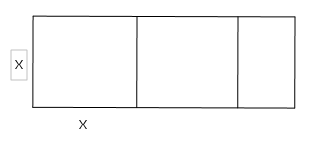The length of a rectangular sheet shown in the figure is 13 cm.

From this sheet two square sheets of maximum size are cut off.

The area of the remaining sheet is 15 sq.cm.

(a) if the width of the sheet is x , what is its breadth of the remaining sheet ?

(b) Forming a second degree equation, find the length and breadth of the remaining sheet.

Get Free Study Materials + 1 Week Free Trial of BrainsPrep Class 10 English Medium Tuition

Qn 5.

A pond of rectangular shape is to be constructed with perimeter 42 m and diagonal length 15 m.

If breadth of the pond is 'x', what is its length?

Form a second degree equation and hence find the length and breadth of the pond.

Get Free Study Materials + 1 Week Free Trial of BrainsPrep Class 10 English Medium Tuition

Qn 6.

When 4 cm is subtracted from each side of a square, area becomes 144 square cm. Form an equation by taking x as the side of larger squire. Find the side of the large square?

Length of a side of the  large square =x , then the length of a side of the small  square=x-4

Get Free Study Materials + 1 Week Free Trial of BrainsPrep Class 10 English Medium Tuition

Qn 7.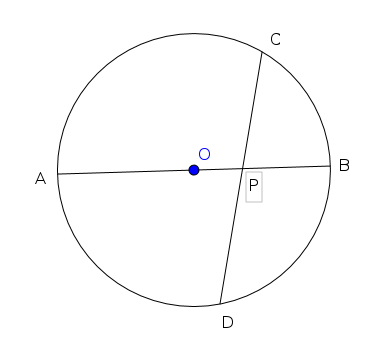In the figure AB is the diametre of the circle. The chord CD cut AB at P.

AB = 16 cm , CD = 14 cm , PC = 6 cm

(a) If PA = x , Find PB .

(b) Find the length of PA.

Get Free Study Materials + 1 Week Free Trial of BrainsPrep Class 10 English Medium Tuition

Qn 8.

When  breadth is increased by 2 cm and length is reduced by 3 cm of a rectangle with perimeter 60 cm, the area of the newly formed rectangle became 210 sq.cm.

(a) if  width of the first rectangle is x , what is its length ?

(b) What is the length of the newly formed rectangle ?

(c) Forming a second degree equation, find the length and breadth of the first rectangle.

Get Free Study Materials + 1 Week Free Trial of BrainsPrep Class 10 English Medium Tuition

Qn 9.

Sum of the first n consecutive natural  numbers is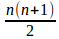. Then, how many natural numbers are to be  added to get a sum 325 ?

Get Free Study Materials + 1 Week Free Trial of BrainsPrep Class 10 English Medium Tuition

Qn 10.

Sum of the squares  of two consecutive even  numbers is 452.

a) If one number is 'x' , then what is the next number ?

b) Form the second degree equation and find the numbers

Get Free Study Materials + 1 Week Free Trial of BrainsPrep Class 10 English Medium Tuition

Qn 11.

Number in the unit place of a two digit number is 3 more than that in the tenth place number. Product of the number and the sum of its digits is 70. What is the number?

Get Free Study Materials + 1 Week Free Trial of BrainsPrep Class 10 English Medium Tuition

Qn 12.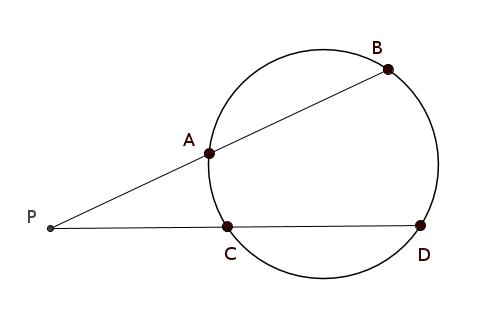In the figure, the chord AB and CD are extended and met at P. If PB = 14 cm, AB = 5 cm, CD = 15 cm, what is the length of PC?

Get Free Study Materials + 1 Week Free Trial of BrainsPrep Class 10 English Medium Tuition

Qn 13.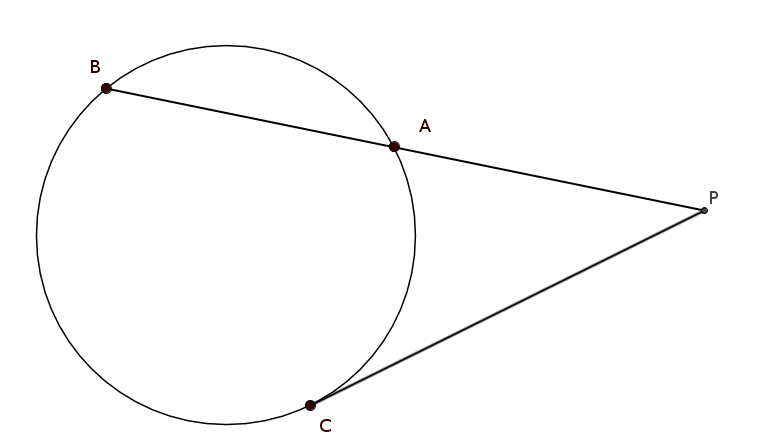In the figu

re, AB = 9 cm, PC= 6 cm, then what is the length of PA?

Get Free Study Materials + 1 Week Free Trial of BrainsPrep Class 10 English Medium Tuition